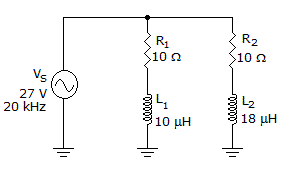# Electronics - RL Circuits - Discussion

Discussion Forum : RL Circuits - General Questions (Q.No. 2)
2.Calculate the voltage dropped across R1 in the given circuit.

14 V
26.8 V
28 V
0 V
Explanation:
No answer description is available. Let's discuss.
Discussion:
11 comments Page 1 of 2.

Vivek yadav said:   3 years ago
What is the voltage drop across L1? Please explain anyone.

Saran said:   4 years ago
R1 is 10K, then how it will be R1=10^2?

Blank said:   5 years ago
FOR DC VOLTAGE inductor treated as a short circuit?

KIRAN V said:   6 years ago
I think voltage is same at any point in a parallel circuit.

Prasanna kumar said:   7 years ago
Voltage across resistor, R1= Resistance value * Supply voltage/Total resistance.
VR1 = R1*Vs/ (sqrt(R1^2+(2*pi*f*L)^2).
VR1 = 10*27/(sqrt(10^2 + (2*pi*20*10^3*10*10^- 6)^2).
= 26.78V.

Dipanjan said:   8 years ago
XL1 = 2*pi*f*L=2*3.14*20*10^3*10^-5 = 1.256 ohms.

Z1 = sqrt[(R1^2)+(XL1)^2] = sqrt(10^2+1.256^2) = 10.0786 ohms.

Voltage dropped across R1 = Vs*(R1/Z1) = 27*(10/10.0786) = 26.8 V.

Amit kumar sahoo said:   9 years ago
Find REACTANCE XL using XL = 2*pi*f*L.
Find total impedance Z = (R^2+XL^2)^1/2.

Using voltage divider formula find voltage across R.
VOLTAGE ACROSS R = TOTAL VOLTAGE*(R/Z).

XL for L1 => 2*pi*20 000*10x10^-6 = 1.256 ohms

Sqr(R1^2+XL^2) = sqrroot(10^2+1.256^2) = 10.0786 ohms

And use voltage divider Vs(R1/R total) = 27(10/10.0786) = 26.789 V or 26.8 V